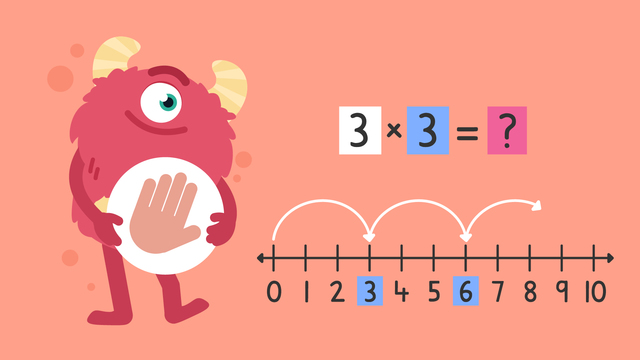# Multiplication on a Number Line — Let's Practice!Rating

Ø 4.0 / 6 ratings
The authorsTeam Digital
Multiplication on a Number Line — Let's Practice!
CCSS.MATH.CONTENT.3.OA.C.7

## Basics on the topicMultiplication on a Number Line — Let's Practice!

Today we are practicing multiplication on a number line with Razzi! This video contains examples to help you further practice and grow confident in this topic.

### TranscriptMultiplication on a Number Line — Let's Practice!

Razzi says get these items ready, because today we're going to practice multiplication on a number line. It's time to begin! Solve three times four using the number line. Pause the video to work on the problem, and press play when you are ready to see the solution! Starting from zero, do three jumps of four each time, to land on twelve. Did you also get twelve? Let's tackle the next problem! Solve seven times two using the number line. Pause the video to work on the problem, and press play when you are ready to see the solution! Starting from zero, do seven jumps of two each time, to land on fourteen. Did you also get fourteen? Let's solve the next one! Solve three times three using the number line. Pause the video to work on the problem, and press play when you are ready to see the solution! Starting from zero, do three jumps of three each time, to land on nine. Did you also get nine? Let's tackle the final problem! Solve two times five using the number line. Pause the video to work on the problem, and press play when you are ready to see the solution! Starting from zero, do two jumps of five each time, to land on ten. Did you also get ten? Razzi had so much fun practicing with you today! See you next time!

## Multiplication on a Number Line — Let's Practice! exercise

Would you like to apply the knowledge you’ve learned? You can review and practice it with the tasks for the video Multiplication on a Number Line — Let's Practice!.